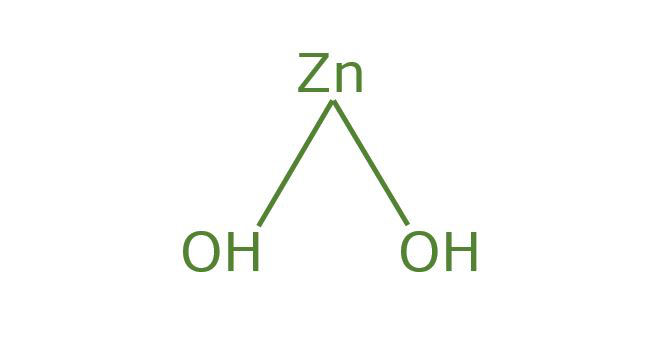GeeksforGeeks App
Open AppBrowser
Continue

# Zinc Hydroxide Formula – Structure, Properties, Uses, Sample Questions

Zinc is a chemical element with the symbol Zn and atomic number 30. Zinc is a slightly brittle metal at room temperature, and when it is deoxidized, it acquires a silvery-gray color. It is the first element in group 12 (IIB) of the periodic table. Hydroxide is any compound containing one or more groups, containing one atom, which is combined with each of the oxygen and hydrogen, and functions as a negatively charged ion. The positive charge portion of the compound may typically be an organic group (e.g. Guanidine or tetramethylammonium), but is generally an ion of metal (e.g., sodium, magnesium, or aluminum).

### What is Zinc Hydroxide?

Zinc hydroxide Zn(OH)2 is an inorganic compound. It also occurs naturally as three rare minerals: wülfingite (orthorhombic), ashoverite, and steatite (both tetragonal).

Zinc hydroxide (and zinc oxide) is amphoteric, like hydroxides of other metals such as lead, aluminum, beryllium, tin, and chromium. Therefore, it is easily soluble in alkaline solutions such as sodium hydroxide as well as in dilute solutions of strong acids such as HCl.

### Zinc Hydroxide Formula

Zinc hydroxide exists on Earth as a rare natural mineral. It is also an amphoteric white solid that is soluble in solutions of strong acids or bases. It occurs in the form of three rare earth minerals: ashoverite, wulfenite, etc. Its formula is Zn(OH)2.

### Structure of Zinc Hydroxide

The chemical formula of zinc hydroxide or formula of zinc hydroxide: Zn(OH)2. It exists in tetragonal or orthorhombic form. Below shown is the general structural representation of zinc hydroxide:Structure of Zinc Hydroxide

### Preparation of Zinc Hydroxide

• A small amount of sodium hydroxide solution is prepared by adding to a solution of any zinc salt.

Zn2+ + 2 OH → Zn(OH)2

• Zn2+ forms ions of high concentrations of water and tetra aqua ions at a low moisture concentration, and this reaction can be written in the form of aqueous ion aqueous ions, and the reaction with hydroxides It can be written in the form of aqueous ions. The donation of the proton is shown below:

Zn2+(OH2)4 (aq) + OH (aq) → Zn2+(OH2)3OH (aq) + H2O (l)

When sodium hydroxide is added, the sodium hydroxide remains dissolved when sodium hydroxide is added. Colorless Ion Zinc Water:

Zn(OH)2 + 2 OH → Zn (OH)42-

The ions are naturally surrounded by water ligands, so zinc is dissolved in the water. The excess sodium hydroxide is added to the solution, and the hydroxide ions reduce the composite to two rates and dissolve. When ammonia is added to the exceeded, the equilibrium that gives a hydroxide ion is formed; The formation of the hydroxide ions causes a reaction similar to sodium hydroxide, which is difficult to induce the formation of the charging +2 complex together with the coordination number 4 having ammonia ligands.

### Properties of Zinc Hydroxide

Physical Properties of Zinc Hydroxide:

• The density of zinc hydroxide is 3.05 g/cm3.
• The molar mass of zinc hydroxide is 99.424 g/mol.
• The melting point of zinc hydroxide is 125° C (257° F).
• The appearance is dull white flocculent precipitate and odorless.
• The valency of Zn in zinc hydroxide is 2 and the oxidation state is +2.
• And the pH of zinc hydroxide is 8.88.
• The zinc hydroxide is slightly soluble in water and insoluble in alcohol.

Chemical Properties of Zinc Hydroxide:

• Aluminum reacts with zinc hydroxide solution to form a white precipitate and dissolves in reagent excess to form an Al(OH)4 complex indicating the presence of aluminum.

2Al3++ (aq)+ 3Zn(OH)2 (aq) → 2Al(OH)3 (s) + 3Zn

• Zinc cations react with hydrogen sulfide in the presence of ammonia, and ammonium chloride forms a white precipitate of zinc sulfide. soluble in acids.

Zn2+(aq) + S2 → ZnS

### Uses of Zinc Hydroxide

• It is used as an adsorbent in medicine and also as a fixative aid in dressings.
• Zinc compounds are applied in large dressings used to absorb blood from wounds after surgery.
• It is useful for acting as an intermediate in industrial processes for pesticides and pigments.

### Sample Questions

Question 1: Is the zinc hydroxide a salt?

Under normal conditions, zinc salts dissociate to form zinc ions, which combine with two hydroxide ions in sodium hydroxide solution to form zinc hydroxide. These properties of zinc hydroxide are widely used to detect the presence of zinc ions in the solution.

Question 2: Is zinc hydroxide soluble or insoluble?

It is well established that zinc hydroxide is slightly soluble in water, becoming more soluble as the pH is either lowered or raised.

Question 3: What does zinc hydroxide consist of?

Zinc hydroxide can be prepared by reacting zinc chloride or zinc sulfate with sodium hydroxide. Zinc hydroxide did not dissolve using dilute sodium hydroxide solution.

Question 4: Is zinc hydroxide acidic?

Zinc hydroxide is the inorganic compound with the chemical formula Zn(OH)2. It is amphoteric in nature and is soluble in both strong base and acid solutions.

Question 5: Why is ZnO used as a pigment?